Anzeige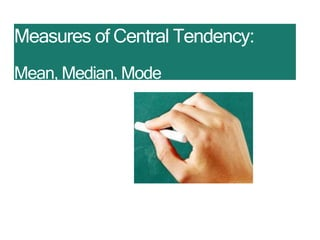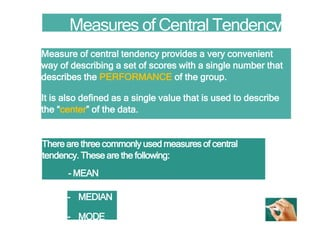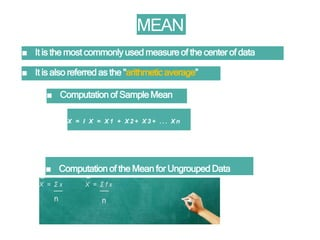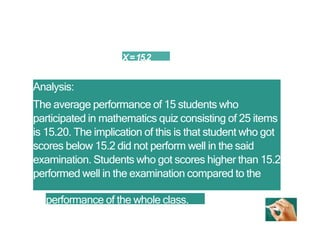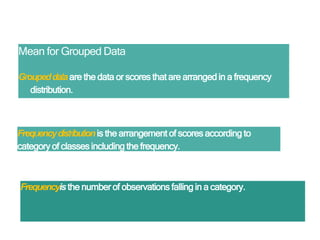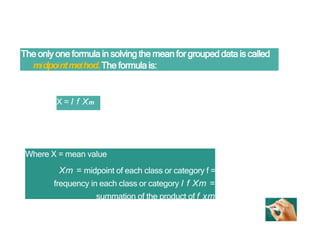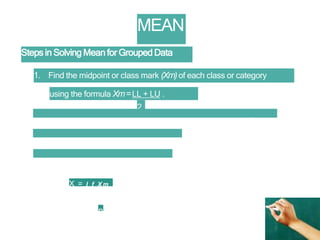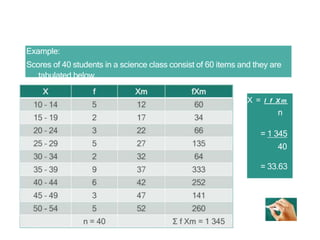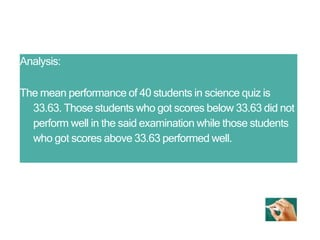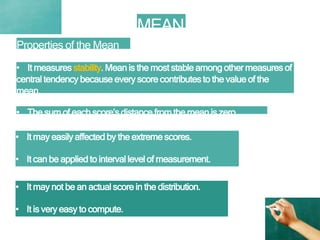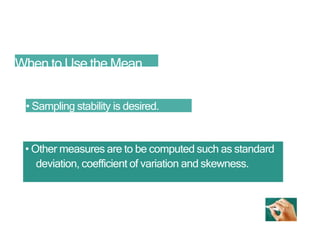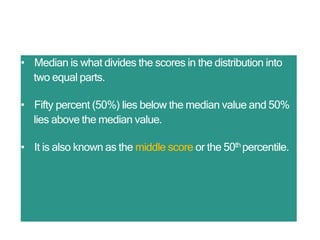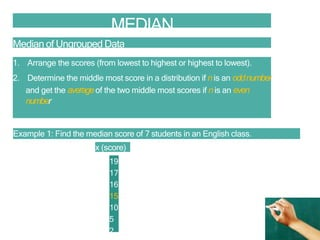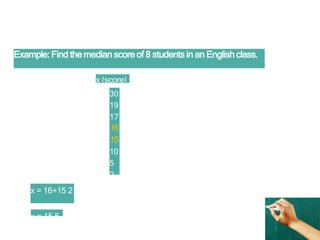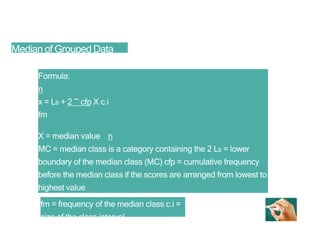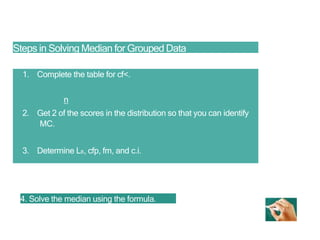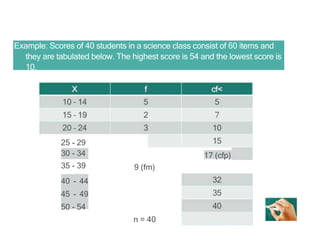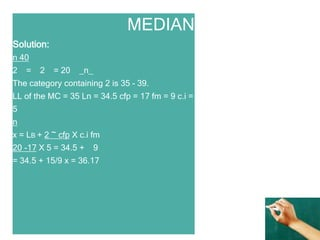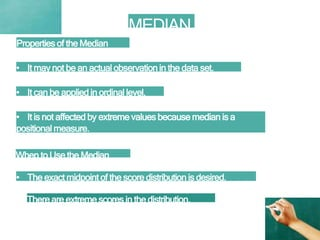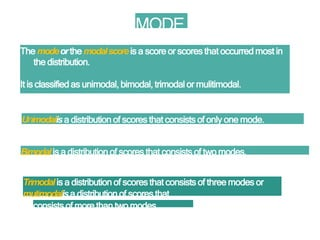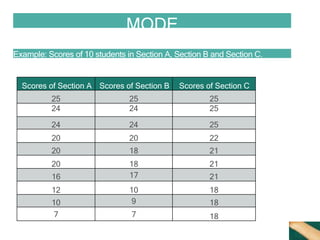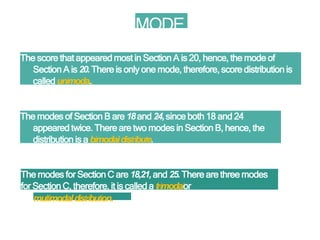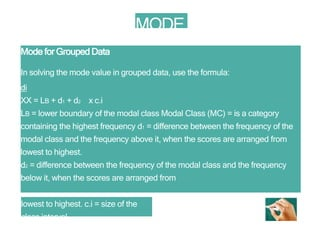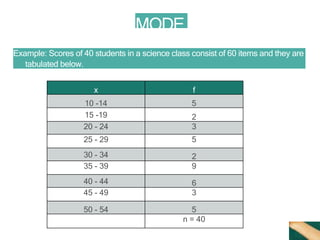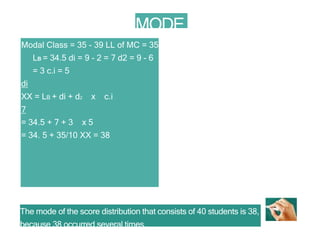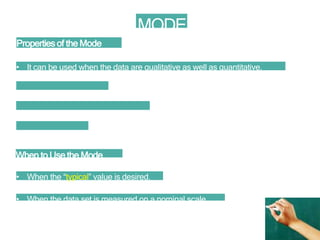1 von 27
Anzeige

### measures of centraltendency.ppt

1. Measures of Central Tendency: Mean, Median, Mode
2. Measures of Central Tendency Measure of central tendency provides a very convenient way of describing a set of scores with a single number that describes the PERFORMANCE of the group. It is also defined as a single value that is used to describe the “center” of the data. There are three commonly used measures of central tendency. These are the following: - MEAN - MEDIAN - MODE
3. MEAN ■ It is the most commonlyused measure of the center of data ■ It is also referred as the "arithmetic average” ■ Computation of Sample Mean X = I X = X 1 + X 2 + X 3 + ... X n ■ Computation of the Mean for Ungrouped Data
4. X=15.2 Analysis: The average performance of 15 students who participated in mathematics quiz consisting of 25 items is 15.20. The implication of this is that student who got scores below 15.2 did not perform well in the said examination. Students who got scores higher than 15.2 performed well in the examination compared to the performance of the whole class.
5. Mean for Grouped Data Groupeddataare the data or scores that are arranged in a frequency distribution. Frequencydistribution is the arrangement of scores according to category of classes including the frequency. Frequencyisthe number of observations falling in a category.
6. The only one formula in solving the mean for grouped data is called midpointmethod.The formula is: X = I f Xm Where X = mean value Xm = midpoint of each class or category f = frequency in each class or category I f Xm = summation of the product of f xm
7. MEAN Steps in Solving Mean for Grouped Data 1. Find the midpoint or class mark (Xm)of each class or category using the formula Xm=LL + LU . 2 2. Multiply the frequency and the corresponding class mark fxm. 3. Find the sum of the results in step 2. 4. Solve the mean using the formula X = I f X m n
8. Example: Scores of 40 students in a science class consist of 60 items and they are tabulated below. X = I f X m n = 1 345 40 = 33.63
9. Analysis: The mean performance of 40 students in science quiz is 33.63. Those students who got scores below 33.63 did not perform well in the said examination while those students who got scores above 33.63 performed well.
10. MEAN Properties of the Mean • It measures stability. Mean is the most stable among other measures of central tendency because every score contributes to the value of the mean. • The sum of each score’s distance from the mean is zero. • It may easily affected by the extreme scores. • It can be applied to interval level of measurement. • It may not be an actual score in the distribution. • It is very easy to compute.
11. When to Use the Mean • Sampling stability is desired. • Other measures are to be computed such as standard deviation, coefficient of variation and skewness.
12. • Median is what divides the scores in the distribution into two equal parts. • Fifty percent (50%) lies below the median value and 50% lies above the median value. • It is also known as the middle score or the 50th percentile.
13. MEDIAN Median of Ungrouped Data 1. Arrange the scores (from lowest to highest or highest to lowest). 2. Determine the middle most score in a distribution if nis an oddnumber and get the averageof the two middle most scores if nis an even number Example 1: Find the median score of 7 students in an English class. x (score) 19 17 16 15 10 5 2
14. Example: Find the median score of 8 students in an English class. x (score) 30 19 17 16 15 10 5 2 x = 16+15 2 x = 15.5
15. Median of Grouped Data Formula: n x = LB + 2 ~ cfp X c.i fm X = median value n MC = median class is a category containing the 2 LB = lower boundary of the median class (MC) cfp = cumulative frequency before the median class if the scores are arranged from lowest to highest value fm = frequency of the median class c.i = size of the class interval
16. Steps in Solving Median for Grouped Data 1. Complete the table for cf<. n 2. Get 2 of the scores in the distribution so that you can identify MC. 3. Determine LB, cfp, fm, and c.i. 4. Solve the median using the formula.
17. Example: Scores of 40 students in a science class consist of 60 items and they are tabulated below. The highest score is 54 and the lowest score is 10. 25 - 29 30 - 34 35 - 39 40 - 44 45 - 49 50 - 54 9 (fm) n = 40 17 (cfp)
18. MEDIAN Solution: n 40 2 = 2 = 20 _n_ The category containing 2 is 35 - 39. LL of the MC = 35 Ln = 34.5 cfp = 17 fm = 9 c.i = 5 n x = LB + 2 ~ cfp X c.i fm 20 -17 X 5 = 34.5 + 9 = 34.5 + 15/9 x = 36.17
19. MEDIAN Properties of the Median • It may not be an actual observationin the data set. • It can be applied in ordinal level. • It is not affected by extreme values because median is a positional measure. When to Use the Median • The exact midpoint of the score distribution is desired. There are extreme scores in the distribution.
20. MODE The modeorthe modalscoreis a score or scores that occurred most in the distribution. It is classified as unimodal, bimodal, trimodal or mulitimodal. Unimodalisa distribution of scores that consists of only one mode. Bimodalis a distribution of scores that consists of two modes. Trimodalis a distribution of scores that consists of three modes or multimodalisa distribution of scores that consists of more than two modes.
21. MODE Example: Scores of 10 students in Section A, Section B and Section C. Scores of Section A Scores of Section B Scores of Section C 25 25 25 24 24 25 24 24 25 20 20 22 20 18 21 20 18 21 16 17 21 12 10 18 10 9 18 7 7 18
22. MODE The score that appeared most in Section A is 20, hence, the mode of Section A is 20.There is only one mode, therefore, score distribution is called unimoda.. The modes of Section B are 18and 24,since both 18 and 24 appeared twice. There are two modes in Section B, hence, the distribution is a bimodaidistribute. The modes for Section C are 18,21,and 25.There are three modes for Section C, therefore, it is called a trimodaior multimodaldistribution.
23. MODE Mode for Grouped Data In solving the mode value in grouped data, use the formula: di XX = LB + d1 + d2 x c.i LB = lower boundary of the modal class Modal Class (MC) = is a category containing the highest frequency d1 = difference between the frequency of the modal class and the frequency above it, when the scores are arranged from lowest to highest. d2 = difference between the frequency of the modal class and the frequency below it, when the scores are arranged from lowest to highest. c.i = size of the class interval
24. MODE Example: Scores of 40 students in a science class consist of 60 items and they are tabulated below. x f 10 -14 5 15 -19 2 20 - 24 3 25 - 29 5 30 - 34 2 35 - 39 9 40 - 44 6 45 - 49 3 50 - 54 5 n = 40
25. MODE Modal Class = 35 - 39 LL of MC = 35 LB = 34.5 di = 9 - 2 = 7 d2 = 9 - 6 = 3 c.i = 5 di XX = LB + di + d2 x c.i 7 = 34.5 + 7 + 3 x 5 = 34. 5 + 35/10 XX = 38 The mode of the score distribution that consists of 40 students is 38, because 38 occurred several times.
26. MODE Properties of the Mode • It can be used when the data are qualitative as well as quantitative. • It may not be unique. • It is affected by extreme values. • It may not exist. When to Use the Mode • When the “typical” value is desired. • When the data set is measured on a nominal scale.
Anzeige